# Non-linear dynamics

Many dynamical systems (cf. Dynamical system) are described by difference equations (mappings)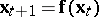, where, or by autonomous systems of differential equations (cf. Autonomous system)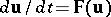, where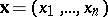and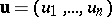. Ifis a non-linear function of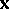, the discrete dynamical system is called non-linear. Piecewise-linear systems are also regarded as being non-linear. A simple example is the logistic mapping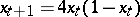. If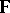is a non-linear function of, one calls the system of differential equations non-linear. A typical example is the pendulum described by,, whereis the displacement and. For small displacements the differential equation reduces to a linear differential equation (cf. also Linear differential operator). For the non-linear equation the amplitude depends on, whereas for the linear equation the amplitude is independent of.
Linear superposition no longer holds for non-linear dynamical systems. The behaviour of solutions of finite-dimensional non-linear difference and differential equations is much more complicated than that of finite-dimensional linear systems. For a linear system, whereis an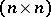-matrix with constant coefficients, the solution of the initial-value problem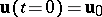exists for. Among non-linear systems one finds the behaviour that the solution only exists for finite times. An example is the differential equation. The solution of the initial-value problem has a singularity at a finite time. Autonomous non-linear dynamical systems in the plane,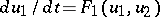,can show limit cycle behaviour (cf. Limit cycle). An example is the van der Pol equation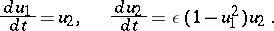For, autonomous systems of first-order ordinary differential equations can show so-called chaotic behaviour (cf. Chaos; Strange attractor). Moreover, the attractor can have a non-integer fractal dimension (capacity and Hausdorff dimension). One of the tasks in non-linear dynamics is to classify the attractors (fixed points, limit cycles, tori, strange attractors). In a non-linear dynamical system, the domain of attraction can have a fractal boundary. Furthermore, certain particular solutions need not be included in the general solution. Most non-linear dynamical systems cannot be solved explicitly. Thus, qualitative methods are applied to study them (cf. Qualitative theory of differential equations). One such method is the Poincaré section. Here, the non-linear differential equation is mapped to a discrete non-linear system. The study of non-linear discrete systems in the complex domain leads to Julia and Mandelbrot sets (cf. Julia set). Non-linear finite-dimensional autonomous systems, whereis an analytic function, and non-linear discrete systems, whereis an analytic function, can be linearized via Carleman linearization to infinite-dimensional linear dynamical systems.